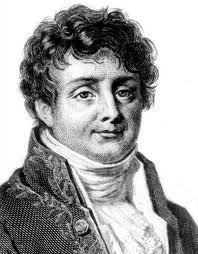• 1768 March 21
(b.) -
1830 May 16
(d.)

#### Bio/Description

Fourier left an unfinished work on determinate equations which was edited by Claude-Louis Navier and published in 1831. This work contains much original matter ? in particular, there is a demonstration of Fourier's theorem on the position of the roots of an algebraic equation. Joseph Louis Lagrange had shown how the roots of an algebraic equation might be separated by means of another equation whose roots were the squares of the differences of the roots of the original equation. Fran?ois Budan, in 1807 and 1811, had enunciated the theorem generally known by the name of Fourier, but the demonstration was not altogether satisfactory. Fourier's proof is the same as that usually given in textbooks on the theory of equations. The final solution of the problem was given in 1829 by Jacques Charles Fran?ois Sturm.
• Date of Birth:

1768 March 21
• Date of Death:

1830 May 16
• Gender:

Male
• Noted For:

Best known for initiating the investigation of Fourier series and their applications to problems of heat transfer and vibrations
• Category of Achievement: